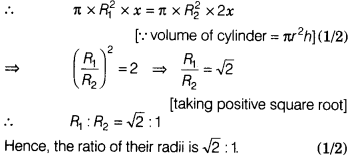# Two circular cylinders of equal volume have their heights in the ratio 1:2

Two circular cylinders of equal volume have their heights in the ratio 1:2. Find the ratio of their radii.

Let the heights of circular cylinder be x units, 2x units and their radii be fi, R2 respectively.
Since, volume of both cylinders are equal.